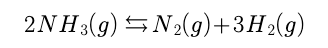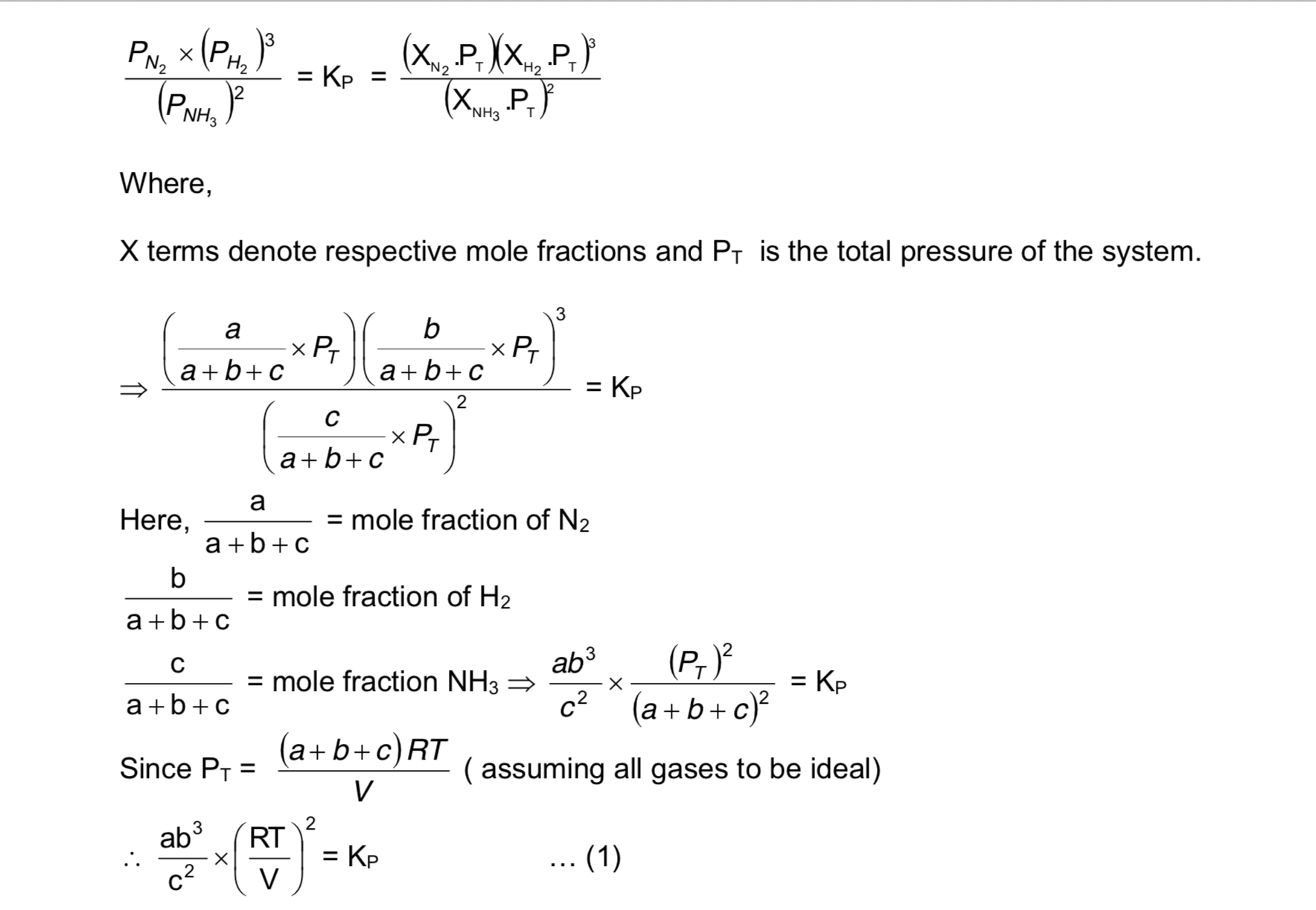# The Le–Chatelier’s Principle - Chemical Equilibrium

The Le–Chatelier’s Principle

This principle, which is based on the fundamentals of a stable equilibrium, states that “When a chemical reaction at equilibrium is subjected to any stress, then the equilibrium shifts in that direction in which the effect of the stress is reduced”.

Confused with “stress”. Well by stress here what I mean is any change of reaction conditions e.g. in temperature, pressure, concentration etc.

This statement will be explained by the following example.

Let us consider the reactionLet the moles of N2, H2 and NH3 at equilibrium be a, b and c moles respectively. Since the reaction is at equilibrium,Now, let us examine the effect of change in certain parameters such as number of moles, pressure, temperature etc.

If we increase a or b, the left hand side expression becomes QP ( as it is disturbed from equilibrium) and we can see that QP > KP. The reaction therefore moves backward to make QP = KP.

If we increase c, QP < KP and the reaction has to move forward to revert back to equilibrium.

If we increase the volume of the container (which amounts to decreasing the pressure), QP < KP and the reaction moves forward to attain equilibrium.

If we increase the pressure of the reaction then equilibrium shifts towards backward direction since in reactant side we have got 2 moles and on product side we have got 4 moles. So pressure is reduced in backward direction.

If temperature is increased the equilibrium will shift in forward direction since the forward reaction is endothermic and temperature is reduced in this direction.

However from the expression if we increase the temperature of the reaction, the left hand side increases (QP) and therefore does it mean that the reaction goes backward (since QP > KP)?. Does this also mean that if the number of moles of reactant and product gases are equal, no change in the reaction is observed on the changing temperature (as T would not exist on the left hand side). The answer to these questions is No. This is because KP also changes with temperature. Therefore, we need to know the effect of temperature on both QP and KP to decide the course of the reaction.

Example :

For the reaction

N2(g) +3H2(g) 2NH3(g), H = – xkcal

What will be the effect

1. a) When pressure is increased?
2. b) When temperature is increased?
3. c) What will be the effect on the equilibrium constant when temperature is increased?